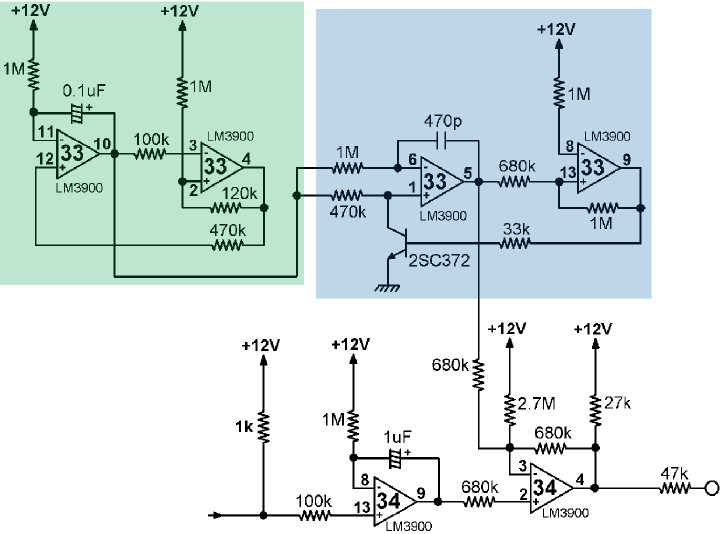# Ufo hit

The sound produced when you hit the UFO ship that sometimes travels at the top of the screen.

The module in the upper left corner (green) is a triangle waveform generator (see AN72, 7.4). According to the application notes if R1=2R2 (and here R1=1M and R2=470K, which is close enough) then the output frequency is (V+ - VBE) / (2 R1 C1 DVO) where DVO is the difference between the trip points of the Schmitt trigger (the second LM3900).

The module in the upper right corner (cyan) is a voltage controlled oscillator (or VCO, see AN72, 8.1) and generates a triangle wave whose frequency is controlled by the output of the first circuit.Unfortunately I don't know how to compute DVO, so this circuit isn't emulated yet. (In the worst case it is always possible to extract the required values from the corresponding sample and use them as the circuit suggest, but that would be simulation more than emulation... not that it is a bad thing anyway...).